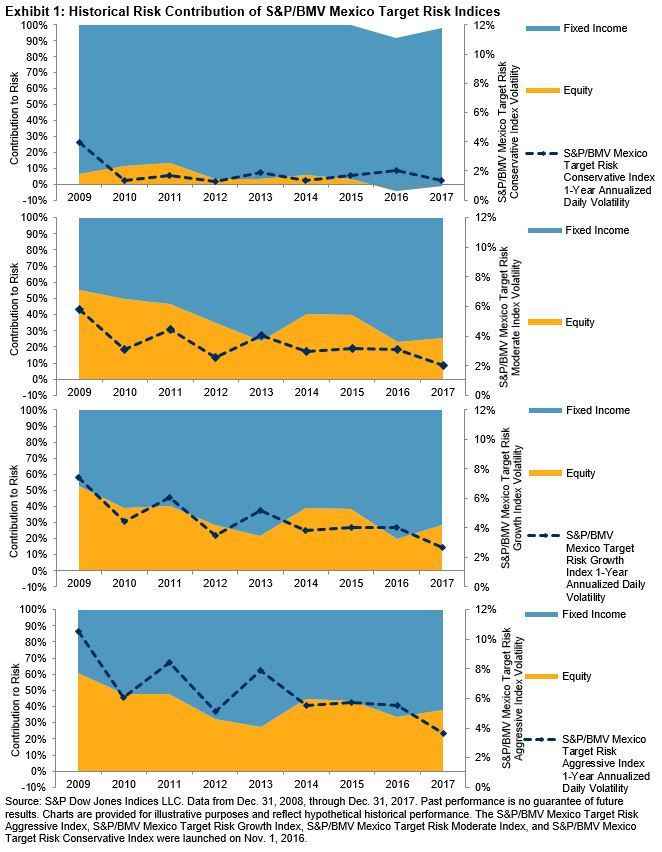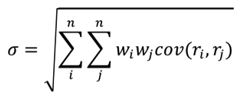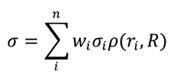# Understanding the Asset Class Risk Contribution of the S&P/BMV Mexico Target Risk Indices

The S&P/BMV Mexico Target Risk Index Series comprises four multi-asset class indices that are designed to serve as benchmarks for the Mexican pension system based on the risk tolerance levels of plan participants. Generally, younger individuals with longer time horizons until retirement will have greater risk tolerance and therefore higher exposure to risky assets such as equities, while older individuals will allocate to more conservative assets such as short-term nominal and inflation-linked bonds. As outlined in our paper, “Benchmarking Lifecycle Investment Strategies: Introducing the S&P/BMV Mexico Target Risk Indices,” we used liquid, investable indices to represent each asset class.

Designed to represent investment strategies with varying levels of risk appetite, the indices allocate across various asset classes to meet their respective risk target. Therefore, it is important to understand the sources of portfolio risk. In this blog, we decompose the risk of each portfolio by asset class so that we can identify and attribute the portion of realized risk coming from each index.

In order to assess the contribution of each asset class to portfolio risk, we first calculate the weighted covariance matrix of all the indices representing each asset class in the index series. As seen in the equations in the footnote, the contribution of a given asset class to portfolio volatility is computed by the correlation between that asset class and the index portfolio multiplied by the volatility of the asset class in question and its weight in the index portfolio. Correlation and volatility are estimated using daily returns over one-year periods from 2009 through 2017.

We then get the percentage of contribution to total portfolio risk by dividing the asset class (or the index) contribution by the total portfolio volatility. The sum of all the contributions must be equal to the total portfolio risk and the sum of the asset class percentages of contributions to portfolio risk must be equal to 1 (or 100%).

Exhibit 1 charts the realized portfolio risk of each S&P/BMV Mexico Target Risk Index, the risk contribution to the index for each asset class, and the percentage of contribution to the total risk for each asset class on an annual basis. For example, in 2017, the total risk of the S&P/BMV Aggressive Target Risk Index was 3.65%, of which 1.36% was from the equity portion, contributing to 38% of the overall risk. The contribution to portfolio risk by the equity portion rose after declining to 34% in 2016.As we noted in our paper, there are periods, such as 2013, when the aggressive portfolio underperformed its growth, moderate, and conservative counterparts due to asset allocation mix. A close examination of asset class contribution to risk provides some insight. For example, in 2013, the biggest contributor to the total risk of the S&P/BMV Mexico Target Risk Aggressive Index came from fixed income, namely the S&P/BMV Sovereign MBONOS 20+ Year Bond Index. At roughly 28% of portfolio total risk, its risk contribution was higher than that of the equity portion (see Exhibit 2).In this blog, we highlighted the importance of understanding the sources of portfolio risk. Asset allocation strategies, such as the S&P/BMV Mexico Target Risk Indices, require decomposition of portfolio risk so that market participants are aware of where the risk is coming from and can assess the impact of each asset class on the overall portfolio.

 To calculate each asset class contribution to risk, we start by defining the portfolio volatility asFrom this equation, the risk contribution of each asset class can be derived asThe posts on this blog are opinions, not advice. Please read our disclaimers.